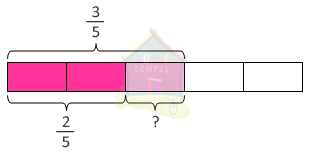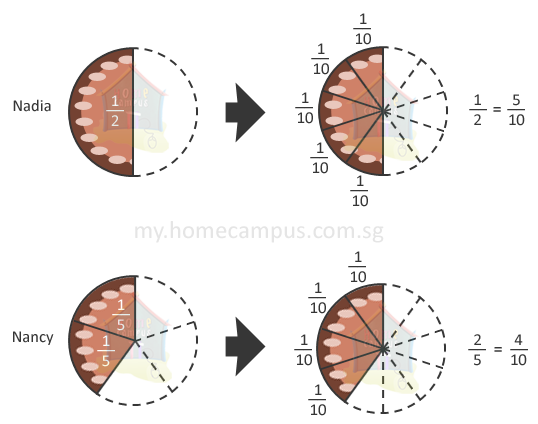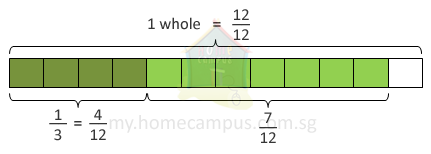## How to Subtract Fractions?

Practice Unlimited Questions

#### How to subtract fractions?

Step 1: Express the fractions using a common denominator. For this you need to find the lowest common denominator.
Step 2: Create equivalent fractions by making the denominator common.
Step 3: Subtract the numerators and leave the denominator as is.
Step 4: Simplify fractions if required.

For better understanding follow the word problem below.

#### 1. Subtract 2 5 from 3 5 .3 5
 2 5

=
 3 - 2 5

=
 1 5

#### 2. Mrs. Chopra had a cake.      She gave 1 2 of the cake to Nadia and 2 5 of the cake to Nancy.      Who received more? How much more?When the fractions to be subtracted have different denominators, we must first change them to their equivalent fractions with the same denominator.

 1 2
=
 5 10

Nancy:
 2 5
=
 4 10

 5 10
is greater than
 4 10

 1 2
 2 5

=
 5 10
 4 10

=
 1 10

 1 10
more of the cake than Nancy.

#### 3. Josh and Halle bought a pizza.      Josh ate 1 3 of the pizza and Halle ate 7 12 of the pizza.      What fraction of the pizza was left?Josh:
 1 3
=
 4 12

Halle:
 7 12

1 −
 4 12
 7 12

=
 12 12
 4 12
 7 12

=
 1 12

 1 12
of the pizza was left.